# Characteristic Impedance

## AC Electric Circuits

• #### Question 1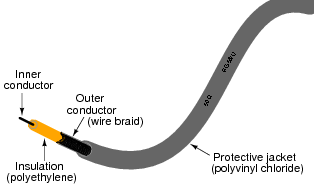Note the “50 ohm” rating printed on the jacket of the cable. What will an ohmmeter register when connected between the inner conductor and shield of the cable? What will an ohmmeter register when connected between opposite ends of either the inner conductor or the shield, from one end of the cable to the other?

• #### Question 2

If a battery and switch were connected to one end of a 10-mile long cable, and two oscilloscopes were used to record voltage at either end of the cable, how far apart in time would those two pulses be, assuming a propagation velocity equal to the speed of light (in other words, the cable has a velocity factor equal to 1.0)?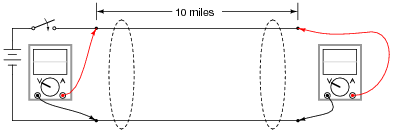• #### Question 3

If a battery and switch were connected to one end of a 10-mile long cable, and two oscilloscopes were used to record voltage at either end of the cable, how far apart in time would those two pulses be, assuming a propagation velocity equal to 69% the speed of light (in other words, the cable has a velocity factor equal to 0.69)?• #### Question 4

What does the “50 ohm” rating of an RG-58/U coaxial cable represent? Explain how a simple cable, with no continuity between its two conductors, could possibly be rated in ohms.

Hint: this “50 ohm” rating is commonly referred to as the characteristic impedance of the cable. Another term for this parameter is surge impedance, which I think is more descriptive.

• #### Question 5

Given the following test circuit, with an oscilloscope used to record current from the battery to the cable (measuring voltage dropped across a shunt resistor), what sort of waveform or pulse would the oscilloscope register after switch closure?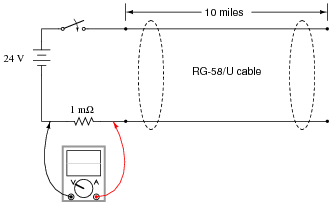• #### Question 6

Given the following test circuit, with an oscilloscope used to record current from the battery to the cable (measuring voltage dropped across a shunt resistor), what sort of waveform or pulse would the oscilloscope register after switch closure?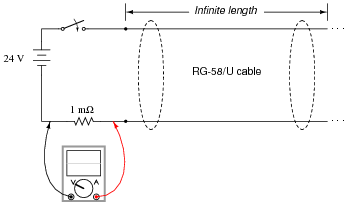• #### Question 7

Suppose this 10-mile-long RG-58/U cable were “terminated” by a resistor with a resistance equal to the cable’s own characteristic impedance: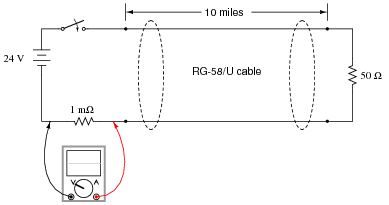What sort of waveform or pulse would the oscilloscope register after switch closure?

• #### Question 8

When a pulse propagates down an “unterminated” cable and reaches an open-circuit, what does it do? Does it simply vanish, or does it go some place else?

• #### Question 9

When a pulse propagates down a cable terminated by a short-circuit, what does it do? Does it simply vanish, or does it go some place else?

• #### Question 10

What will happen if a cable is terminated by a resistor of incorrect value (not equal to the cable’s characteristic impedance)?

• #### Question 11

A two-conductor cable of uniform construction will exhibit a uniform characteristic impedance (Z0) due to its intrinsic, distributed inductance and capacitance: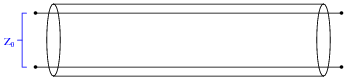What would happen to the value of this characteristic impedance if we were to make the cable narrower, so that the conductors were closer together, all other dimensions remaining the same?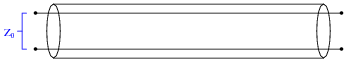• #### Question 12

A two-conductor cable of uniform construction will exhibit a uniform characteristic impedance (Z0) due to its intrinsic, distributed inductance and capacitance:What would happen to the value of this characteristic impedance if we were to make the cable wider, so that the conductors were further apart, all other dimensions remaining the same?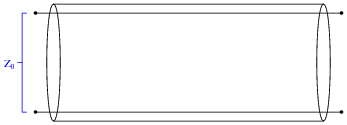• #### Question 13

A two-conductor cable of uniform construction will exhibit a uniform characteristic impedance (Z0) due to its intrinsic, distributed inductance and capacitance:What would happen to the value of this characteristic impedance if we were to shorten the cable’s length, all other dimensions remaining the same?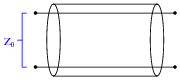• #### Question 14

Suppose we were designing a pair of BJT amplifier circuits to connect to either end of a long two-conductor cable: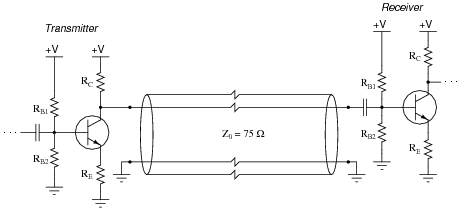How would we choose the component values in each transistor amplifier circuit to naturally terminate both ends of the 75 Ω cable?

• #### Question 15

Suppose we were designing a pair of BJT amplifier circuits to connect to either end of a long two-conductor cable, each end coupled to its respective amplifier through a transformer: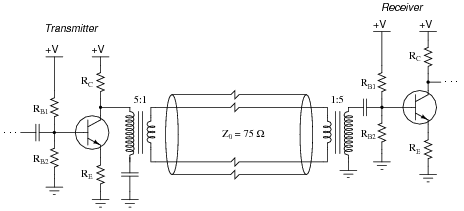How would we choose the component values in each transistor amplifier circuit to naturally terminate both ends of the 75 Ω cable?

• #### Question 16

Some communications networks use cables to not only provide a path for data transmission, but also DC power to energize the circuits connected to the cable.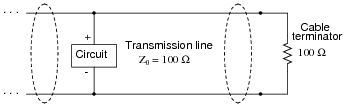However, if we were to terminate the cable as shown, the termination resistor would dissipate a substantial amount of power. This is wasted energy, and would unnecessarily burden the power supply providing DC power to the network cable.

How can we eliminate the problem of power dissipated by the termination resistor in a DC power/signal cable and yet still maintain proper termination to avoid reflected signals?

• #### Question 17

Find a length of coaxial cable and bring it with you to class for discussion. Identify as much information as you can about your piece of cable prior to discussion:

Characteristic impedance
Insulation service (cable tray, conduit, direct burial, etc.)
Type (RG-58, RG-6, etc.)